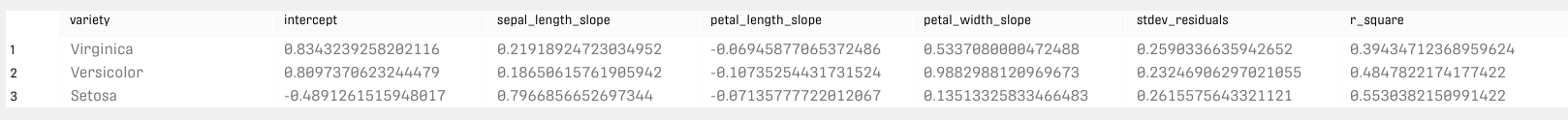# Overview

The Grouped Linear Regression Processor divides the input into several groups, computes and outputs a linear regression model for every group as a row.

# Input

The input data should be suitable for regression forecast and contain one grouping column.

# Output

The processor has two output ports:

• Linear regression models: provides the result of the linear regression for each group. This includes the intercept and the slope values for each independent variable added to the model.
• Group Optimized Input: a group-optimized data structure identical to the input but suitable for re-grouping in similar processors.

# Example

In this example, the input dataset is the iris flower dataset. Each row of the dataset represents an iris flower, including its species and dimensions of its botanical parts, sepal and petal, in centimeters.

Here we're using the iris flower dataset the other way around, which means the variety label here is the grouping column. The dependent variable is the sepal_width.

##The "Group Optimized Input" Result table (second output) is an organized version of the original data table. The grouping column is sorted in alphabetical order.

# Related Articles

Grouped Decision Tree Processor

Grouped Forecast Processor